Next: Acknowledgements Up: Stretching Deca-alanine Tutorial Previous: Viewing SMD trajectories within

Subsections

# Analysis of the SMD trajectory

Launch VMD by typing vmd in a terminal window.

Within VMD select the menu item ExtensionTk Console. A console window should appear with a prompt. The following Tcl/Tk commands are executed from within the TkCon window. We will use the tag » to indicate Tcl commands.

Make sure you are in the correct directory by typing:

 » cd ~/Workshop/10Ala-tutorial/files/

Open the Tcl Output file generated by your SMD simulation, and store its content to a variable mytraj:

 » set infile [open da_smd_tcl.out r] » set mytraj [read $infile] » close$infile

The content of the file da_smd_tcl.out is printed on the screen. You should see three columns:

First column: time (ps)
Second column: extension, or the end to end distance (Å)
Third column: applied force (kcal/mol/Å)

We use the following units: ps for time, Å for distance, kcal/mol for energy.

Assign columns of the mytraj to three separate arrays (lists), by running the following Tcl script:

The script defines three variables, t, z, f, corresponding to each column of mytraj respectively.

Define parameters, v (pulling velocity) and dt (time step of the data):

 » set v 1 » set dt 0.1

Recall that one end of the molecule was constrained to the origin and the other end was constrained to a moving point. The distance between the two constraints was changed from 13 Å to 33 Å with the constant velocity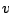. Define an array (list)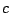representing the distance between the two constraint points at each time step:

 » set c {} » set i 13 » while {$i < 33.1} { lappend c$i; set i [expr $i +$v * $dt] } Plotandcurves using the multiplot plugin within VMD. In the TkCon window type:  » package require multiplot » set plot1 [multiplot -x$t -y $z -plot] »$plot1 add $t$c -plot

Q: The extensiongenerally lags behind, the distance between the two constraints. How big is the lag in this case? What would you do if you want to reduce the lag further?Now plot the force-extension curve:

 » set plot2 [multiplot -x $z -y$f -plot]

Q: Is the force generally positive or negative? Why?The work done on the system during the pulling simulation is:(1)

whereis the pulling velocity.

To calculate the work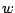by numerical integration, run the following Tcl script:

 » source calcwork1.tcl

The work is stored in a variable (list) called.

If a pulling simulation is performed very slowly, then the process is reversible. The work done during such a reversible pulling is equal to the free energy difference of the system between initial and final states. Thus, a reversible work curve can be considered as an exact PMF. For our system, the reversible pulling speed turns out to be about 0.0001 Å/ps (10000 times slower than the one you used).

The exact PMF obtained from a reversible pulling is stored in the file Fexact.dat. Read the content of the file, and store it in a variable called Fexact, by running the follwong Tcl script:

The array Fexact contains two columns, representing the potenial of mean force (second column) at each extension (first column).

Plot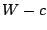in blue together within red:

 » set plot3 [multiplot -x $c -y$w -linecolor blue -plot] » $plot3 add$Fexact(1) $Fexact(2) -linecolor red -plotQ: How do they compare? What is the origin of the difference? Quit VMD:  » quit ## PMF calculation The trajectory you have generated in this session is actually not practical for the PMF calculation because the pulling speed was too high. Besides, you need multiple trajectories to use Jarzynski's equality. Therefore, you will use pre-generated trajectories. Launch VMD by typing vmd in a terminal window. Within VMD select the menu item ExtensionsTk Console. The following commands are all executed from within the TkCon window. Make sure you are in the correct directory by typing:  » cd ~/Workshop/10Ala-tutorial/files/ Ten trajectories obtained from SMD simulations with a pulling speed of 0.01 Å/ps are stored in da.dat. This pulling speed is 100 times slower than the one you used, but still 100 times faster than the reversible speed. Load the trajectories as well as the exact PMF:  » source load-Fexact.tcl » source load-traj.tcl The script load-traj.tcl defines three variable. The arraycontains time steps of the data.and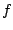are matrices of 10 columns, with each column representing the extension and the applied force for each trajectory, respectively. To plot the extension-time curve for each trajectory, you need to reduce the number of data points plotted for each graph. The following script plotsvsfor a tenth of the data points. Each trajectory is plotted with a different color:  » source plot-extension-time.tcl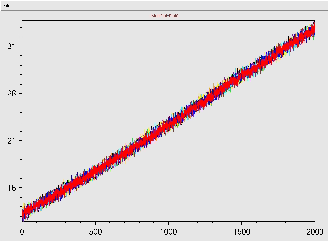And plot the force-extension curve:  » source plot-force-extension.tcl In each figure window, you should see ten overlapping curves, with each curve corresponding to a trajectory.Define parameters: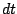, time step;, pulling speed;, temperature (including Boltzmann's constant).  » set dt 0.1 » set v 0.01 » set T 0.6 Define an arrayrepresenting the distance between the two constraint points at each time step:  » set c {} » set i 13 » while {$i < 33.001} {lappend c $i; set i [expr$i + $v *$dt] }

Calculate work for each trajectory by executing the following command:

 » source calcwork2.tcl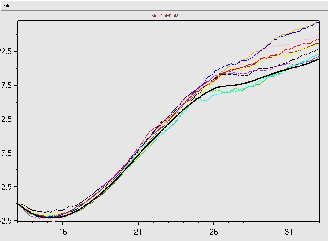Plotvs.together with the exact PMF:

 » source plot-W.tcl

Plot the exact PMF on the same graph:

 » $plot3 add$Fexact(1) $Fexact(2) -linewidth 3 -linecolor black -plot You should see ten work curves and one curve for the exact PMF. The thick black line is the exact PMF. Q: Work done to the system should be on average higher than the PMF. Are all the work curves higher than the PMF, or do you also see some work curves lower than the PMF? Let's now employ Jarzynski's equality: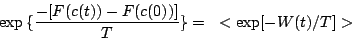(2) or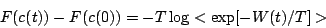(3) The right-hand side can be expanded in terms of so-called cumulants: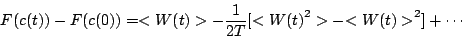(4) where the cumulants up to the second order are shown. Estimate the PMF according to three different formulas: the original formula (exponential average), the first order cumulant expansion formula (average work), and the second order cumulant expansion formula. The following Tcl script will calculate and store the three different estimates in Fexp, F1, F2 variables.  » source cumulants.tcl Plot the three estimates for the PMF together with the exact PMF. Plot the exact PMF, Fexact, in red, the average work (or the first order cumulant expansion),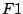, in green, the second order cumulant expansion,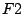, in blue, and the exponential average, Fexp, in black:  » set plot4 [multiplot -plot] »$plot4 add $Fexact(1)$Fexact(2) -linecolor red -plot » $plot4 add$c $F1 -linecolor green -plot »$plot4 add $c$F2 -linecolor blue -plot » $plot4 add$c \$Fexp -linecolor black -plot

Q: Which estimate is the closest to the exact PMF? Does it make sense to you in view of what you learned in the lecture?Quit VMD:

 » quit

For more information on the PMF calculation from SMD simulations, see the paper included (paper.pdf).Next: Acknowledgements Up: Stretching Deca-alanine Tutorial Previous: Viewing SMD trajectories within
tutorial-l@ks.uiuc.edu